Up: Brownian Motion and Focker-Planck Previous: Langevin Equation

Subsections

# Diffusion Approach. Focker-Planck Equation

## Markov Systems

### Time-dependent Distribution Function

In equilibrium thermodynamics we introduced distribution function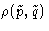: the probability to have momenta around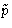and coordinates around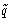is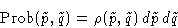• We will study one Brownian particle--one coordinate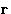and one velocity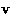• We want a time-dependent solution--we must introduce t
We introduce a time-dependent distribution function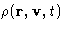--the probability to be in certain place with certain velocity at certain time!

### Transfer Probabilities

If the Brownian motion is the slowest mode in the system, there exists such time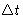that:

1.is large enough, so there are many molecular collisions during this time
2.is small enough, soanddo not change much''
We know the state of our particle at time t:and. What is its state at the time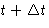?

Assumption:
The state of the system at the momentdepends only on the state at the moment t. The system has short memory.
Definition:
A system satisfying this assumption is called Markov system. We assume Brownian motion to be Markovian.

We introduce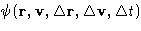--the probability to go from state 1 to state 2:or, introducing the variable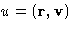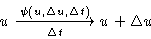The function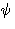is called transfer function. The evolution of Markov system depends only on transfer function and initial state.

### Property of the Transfer Function

Whatever is the state you started from, you will finish at some state with probability 1: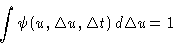(8)

## Chapman-Kolmogorov Equation

What is the probability to find particle at the point u at the time? If it was at the point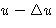at time t, the probability is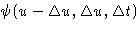. The probability to be atwas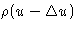. We obtain: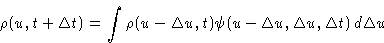(9)
This is called Chapman-Kolmogorov (or master) equation.

## Expansion

Now we will use the fact thatis small. In the left hand side of (9) we have: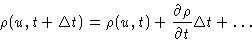In the right hand side (do not forget that u is a vector!):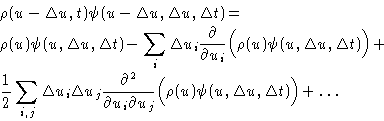Integration:

1.
First term gives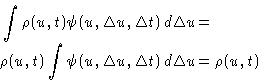2.
Second term gives:3.
Third term gives: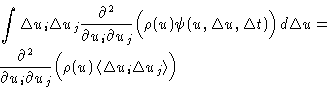## Focker-Planck Equation

We obtained: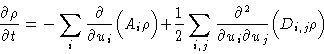(10)
with drift coefficients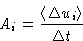and diffusion coefficientsThis is called Focker-Planck equation. It describes diffusion in phase space.

The evolution is described by the moments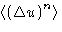. In the Focker-Planck equation we use only the first and second moments. In principle, we can construct an approximation based on higher moments (Kramers, 1940).

## Calculation of Coefficients

For Brownian motion we have:

1.
Linear terms are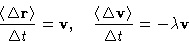2.
Cross terms are zero: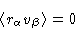3.
Quadratic interm is: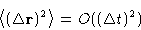4.
Quadratic interm is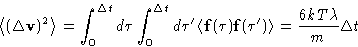Result (summation over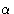&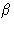is implied):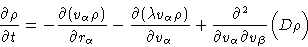with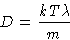## Large Viscosity. Smoluchowski Equation

If viscosity is large, we can assume thatquickly relaxes to equilibrium value: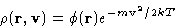Integrating Focker-Planck equation, we can obtain diffusion equation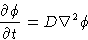Up: Brownian Motion and Focker-Planck Previous: Langevin Equation

© 1997 Boris Veytsman and Michael Kotelyanskii
Sun Nov 2 18:50:28 EST 1997